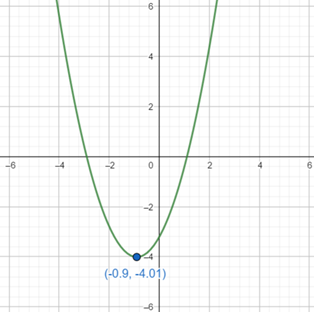# To graph the function using graphing device and find the maximum and minimum value of the function f.### Precalculus: Mathematics for Calcu...

6th Edition
Stewart + 5 others
Publisher: Cengage Learning
ISBN: 9780840068071### Precalculus: Mathematics for Calcu...

6th Edition
Stewart + 5 others
Publisher: Cengage Learning
ISBN: 9780840068071

#### Solutions

Chapter 3.1, Problem 49E

a.

To determine

## To graph the function using graphing device and find the maximum and minimum value of the function f.

Expert Solution

### Explanation of Solution

Given information : Use graphing device to graph the function f(x)=x2+1.79x3.21 .

Graph : Sketch the graph using graphing utility.

Step 1: Press WINDOW button to access the Window editor.

Step 2: Press Y= button.

Step 3: Enter the expression f(x)=x2+1.79x3.21 which is required to graph.

Step 4: Press GRAPH button to graph the function.

The graph is obtained as:Interpretation :

From the above graph, it can be observed that the minimum value is at (4.01)

b.

To determine

### To Find: The exact maximum or minimum value of the function f(x)=x2+1.79x−3.21 and compare it with the above answer.

Expert Solution

The value of minima is f(0.85)=(4.01)

Of course, the result agrees with what our graphing program gave us with the difference that the program rounded the answer to three decimal places.

### Explanation of Solution

Given: The function is f(x)=x2+1.79x3.21

Calculation:

This is a quadratic function with a=1, and b=1.79 . Thus, the maximum or minimum value occurs at

x=b2a=1.7921=1.792=0.895

Since, a>0 , the function has the minimum value

f(0.895)=(0.895)2+1.79(0.895)3.21=4.011025

Of course, the result agrees with what our graphing program gave us with the difference that the program rounded the answer to three decimal places.

### Have a homework question?

Subscribe to bartleby learn! Ask subject matter experts 30 homework questions each month. Plus, you’ll have access to millions of step-by-step textbook answers!